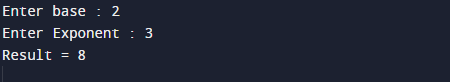C Program to Find Power of a Number

In this tutorial you will learn about the C Program to Find Power of a Number and its application with practical example.

In this tutorial, we will learn to create a C program that will find the power of a given number using C programming.

Prerequisites

Before starting with this tutorial we assume that you are best aware of the following C programming topics:

• C Operators
• C  Loops.
• Basic input/output functions.

Logic behind to find power of any number without using pow() function in C programming.

Example

Input:
take  value of  base : 3
take  value of  exponent : 3
Output:
Input base is : 3
Input exponent : 3

So power of any number basen given as base*base*…..*base (n-times). Here base is called base and n is called the exponent.Here we will write a C program to find the power of a given number.

Program to find power of any number.

In this program we will find power of any given number using for loop. We would first declared and initialized the required variables. Next, we would prompt user to input the number and power of number .Then we will find the power of given number.

OutputNote: The above program will works only if the exponent is a positive integer (number).

In the above program, we have first declared and initialized a set variables required in the program.

• base = it hold the value of base
• expo = it will hold the power
• power= it will hold the result.

Let see the logic of program step by step.

Step 1. Input base and exponents from user, and put  it in two variables  base and expo.
Step 2. Initialize another variable that will store power power=1.
Step 3.within the loop value of i start from 1 to expo, increment loop  by 1 in each iteration. The structure  look like.
Step 4.For every iteration the value of  power  multiply with base.
Step 5.Finally we get the answer in Power.
In this tutorial we understand how to write a program without using Power Function In C to calculate power of a number.

In this tutorial we have learn about the C Program to Find Power of a Number and its application with practical example. I hope you will like this tutorial.